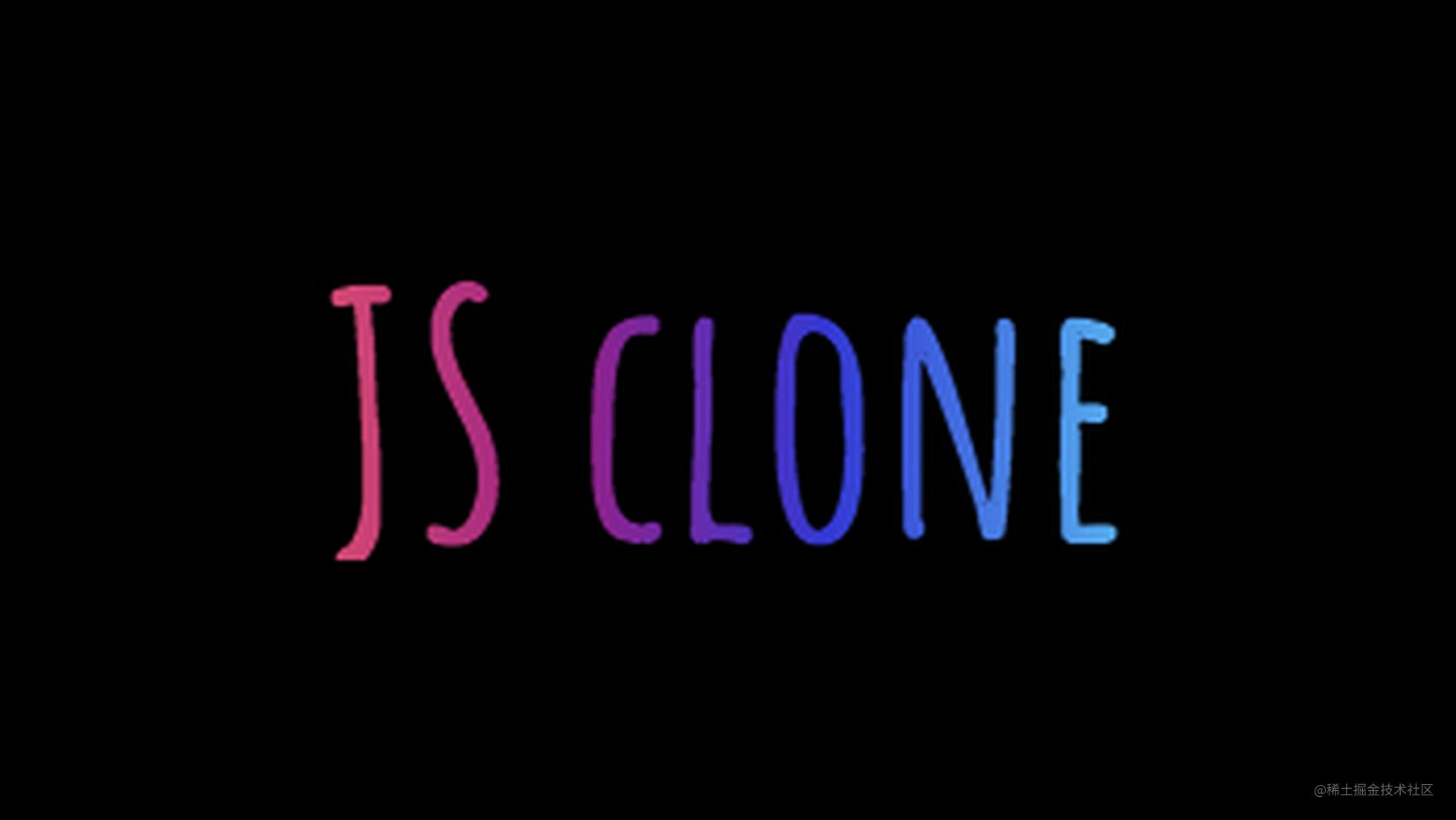# 手撕-JS Clone

js Clone分为浅拷贝和深拷贝，通俗来说，浅拷贝就是数据结构首层不会指向同一个引用，深拷贝就是数据结构所有层级都互不影响。

#### 浅拷贝

##### 1. 万能的`for`循环
``````let a = {
id: 1,
say: (str) => {
console.log(str)
},
other: {
like: ['music', 'code']
}
}

Object.prototype._shallowClone = function() {
let tmp = {}
for (let k in this) {
tmp[k] = this[k]
}
return tmp
}

let a_copy = a._deepClone()

##### 2. 扩展运算符 `...`

``````let a_copy = { ...a }

##### 3. `Object.assign()`

``````let a_copy = Object.assign({}, a)

#### 深拷贝

##### 1. `JSON`
``````Object.prototype._deepClone = function() {
try {
return JSON.parse(JSON.stringify(this))
} catch(err) {
// 处理一些异常
return null
}
}

##### 2. 递归处理

``````function _deepClone(source) {
if (typeof source !== 'object') return source
if (source === null) return null

let tmp = source._isType('Array') ? [] : {}
// 亦可用source.constructor === Array ? [] : {} 来处理

Object.keys(source).forEach(k => {
tmp[k] = (source[k] && typeof source[k] === 'object') ? _deepClone(source[k]) : source[k]
})

return tmp
}

// 检测类型
Object.prototype._isType = function(type) {
return Object.prototype.toString.call(this) === `[object \${type}]`
}

--------------手动华丽分割线（掘金大大考虑编辑器加个分割线？）-------------

##### 3. `MessageChannel`

MessageChanel MDN，关于这个是啥，请大家移步到此MDN链接阅读，这里就不再具体阐述概念了。

``````function _deepClone(source) {
return new Promise((resolve, reject) => {
const { port1, port2 } = new MessageChannel()
port1.onmessage = ({ data }) => resolve(data)
port2.postMessage(source)
})
}

let a_clone

_deepClone(a).then(res => {
a_clone = res
}).catch(err => {
// do some thing
})